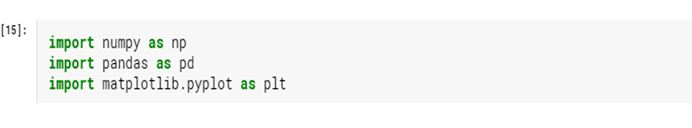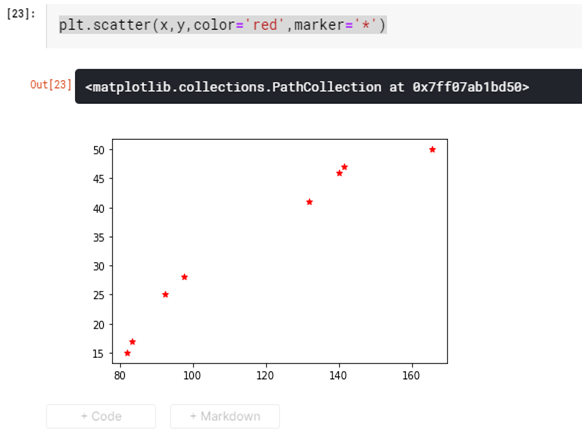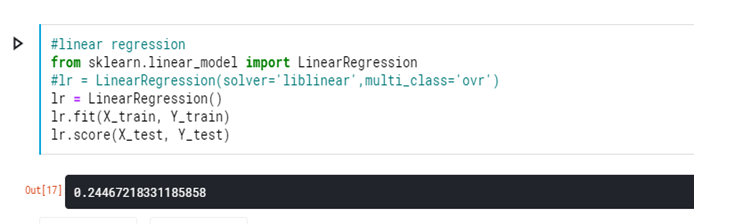Search

# Regression -Scatterplot and ImplementationRegression analysis is a form of predictive modelling technique which investigates the relationship between a dependent and independent variable.

In simple words regression means using the relationship to find the best fit line or the regression equation that can be used to make predictions.

There are many types of regressions such as ‘Linear Regression’, ‘Polynomial Regression’, ‘Logistic regression’ and others but in this blog, we are going to study “Linear Regression” in detail.

Types:

1)Liner Regression

2)Logistic Regression

3)Polynomial Regression

Linear Regression: -

Linear Regression establishes a relationship between dependent variable (Y) and one or more independent variables (X) using a best fit straight line (also known as regression line).

It is represented by an equation :

Y = bX+C,

where Y is a dependent variable

X, is the independent variable, that shows a best fitted straight line(regression curve) having b as the slope of the line and C is intercept.The difference between the liner and multiple linear regression is that the multiple linear regression has more than (>1) one independent variables, whereas the simple linear regression has only one independent variable.

Let’s use the data from the table and create our Scatter plot and linear regression line using http://endmemo.com/statistics/lr.php:How to Create a Scatterplot with a Regression Line in Python

Using Matplotlib: -#Create the data#Create the basic scatterplot# To obtain m(slope) and b (intercept)of linear regression line# Add Linear Regression line to scatterplotAs you all will notice the no. of umbrellas sold increases with the increase in amount of rainfall .So it is a Positive Linear Regression here.

Implementation of Linear Regression in python: -

There are five basic steps when you’re implementing linear regression:

1. Import the packages and classes you need.

2. Provide data to work with and eventually do appropriate transformations.

3. Create a regression model and fit it with existing data.

4. Check the results of model fitting to know whether the model is satisfactory.

5. Apply the model for predictions.

We are going to use the Melbourne database for this Linear regression here below

Import packages and classes

The first step is to import the package numpy and the class LinearRegression from sklearn.linear_model:Read the data to work withThis function fetches the no of records specified within the parathesis()Spilt the Data

Spilt the data into test and Train.Run the model and evaluateI have tried to Explain the Linear Regression. How to scatterplot and its implementation. How the formula for Linear Regression works .